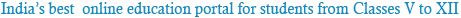### Sample Test Mathematics

1
Between any two rational numbers, there lie :
• 1 two rational number
• 2 No rational number
• 3 infinite rational numbers
• 4 infinite fractions
2
A rhombus can not be drawn if
• 1 one side and one diagonal are given.
• 2 the lenths of the two diagonals are given.
• 3 the length of one diagonal is given.
• 4 None of these.
3
• 1 Natural numbers
• 2 Whole Numbers
• 3 Rational Numbers
• 4 All of these
4
Rational numbers are not closed under :
• 1 Subtraction
• 2 Division
• 4 Multiplication
5
In a linear equation, the highest power of the variableis :-
• 1 One
• 2 two
• 3 Three
• 4 Zero
6
An equation of the form ax + b = c, where a, b and c are numbers, a not equal to 0 and x is the variable; represents a
• 1 linear equation
• 2 linear equation in one variable
• 3 linear equation in two variables
• 4 None of these
7
The sum of the exterior angles of any polygon is:
• 1 180°
• 2 360°
• 3 90°
• 4 60°
8
Which of the following is a regular polygon:
• 1 Isosceles triangle
• 2 Square
• 3 Parallelogram
• 4 Rhombus
9
The opposite angles of a................ are equal.
• 1 Parallelogram
• 2 Rhombus
• 3 Trapezium
• 4 None of these
10
• 1 two
• 2 three
• 3 four
• 4 five

Powered by: iVerticals Web Technology Pvt Ltd